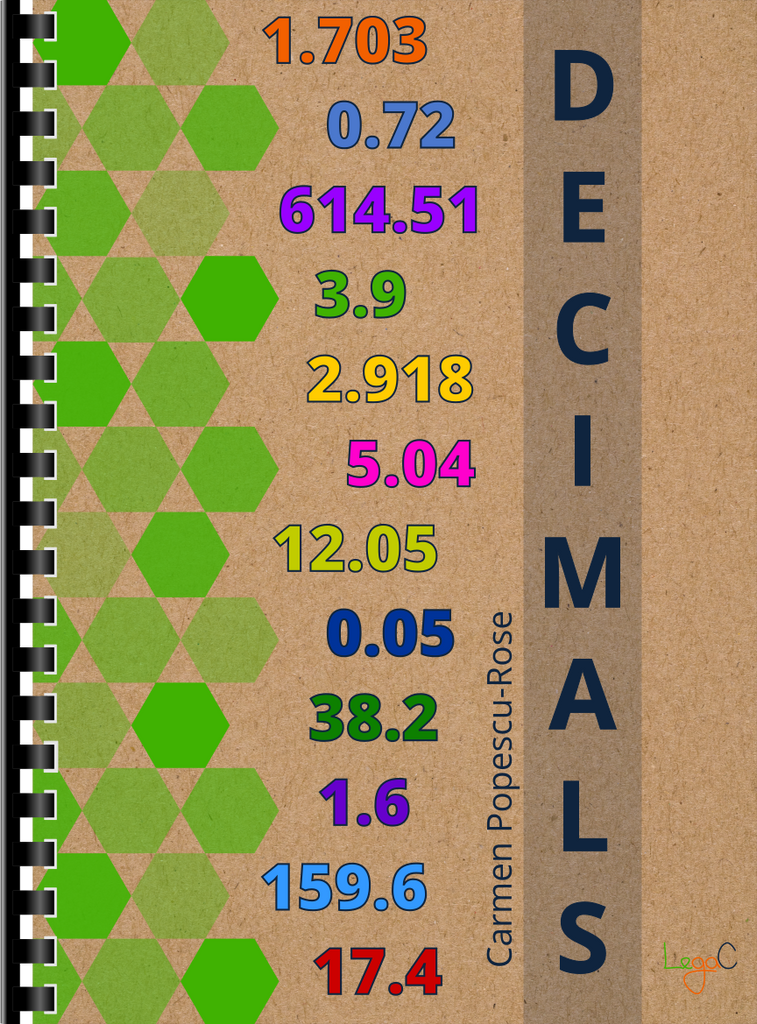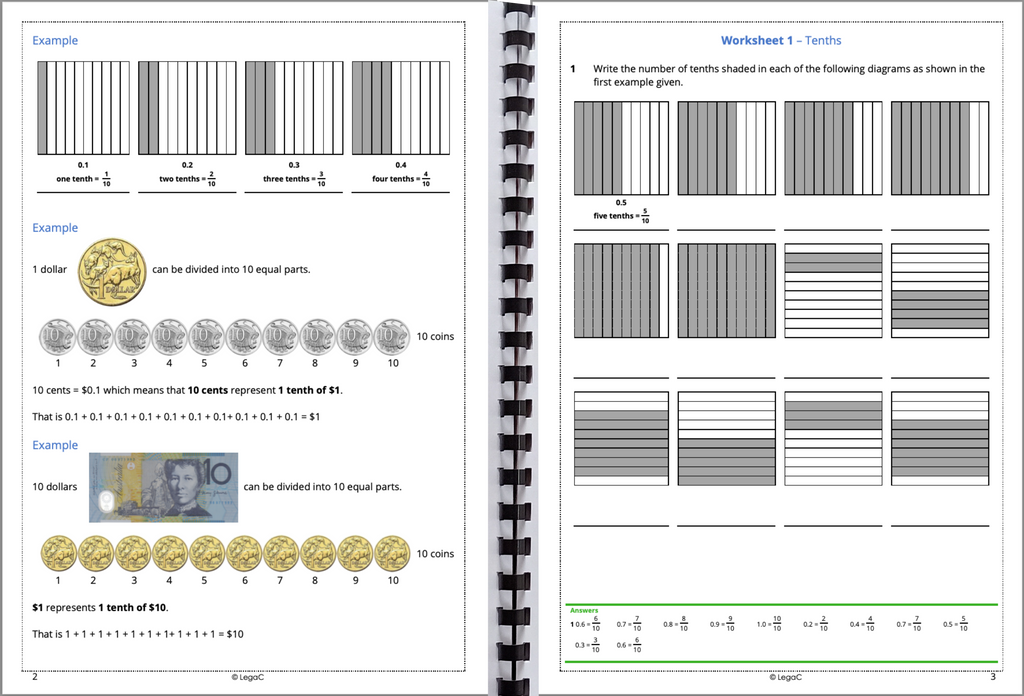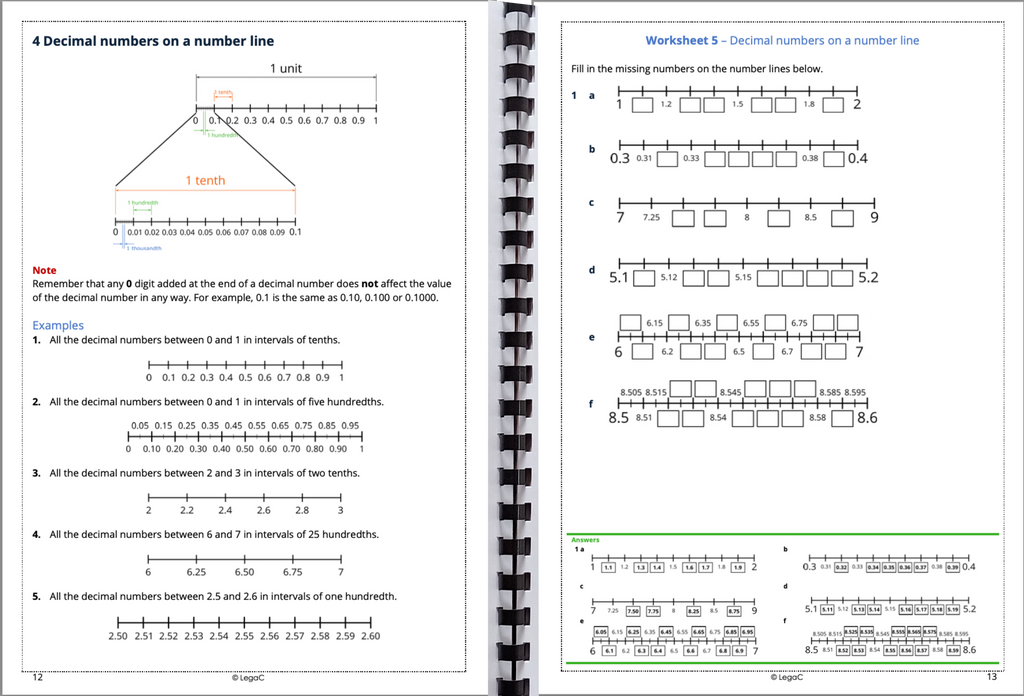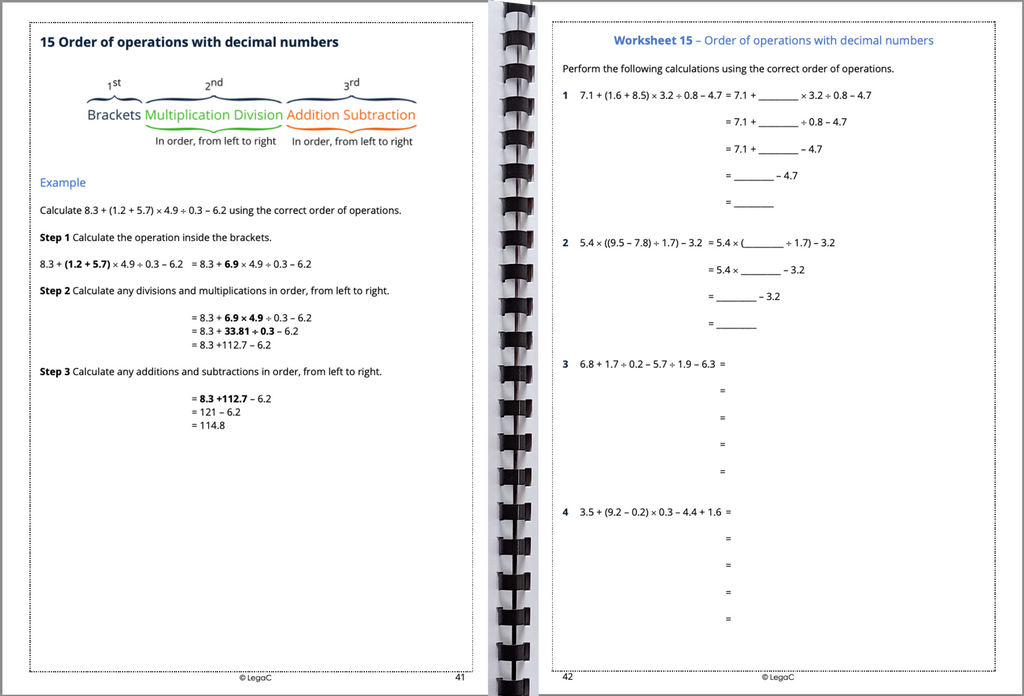Double click for enlarge# Decimals Workbook

Availability: In stock
Quantity:
\$25.00
This 50-page book covers
* Place value for decimal numbers
* Rounding and comparing decimal numbers
* Ordering decimal numbers
* Addition, subtraction, multiplication and division of decimal numbers
* Multiplying and dividing decimal numbers by powers of 10
* Order of operations with decimal numbers
There are 15 worksheets, two Review sets and Problem solving questions.

(0) Items
Items 0
Subtotal \$0.00
To Top